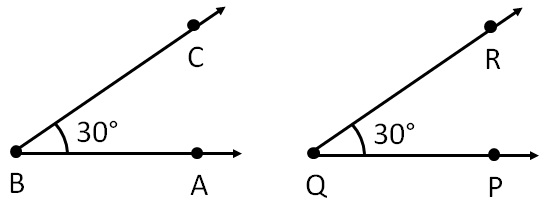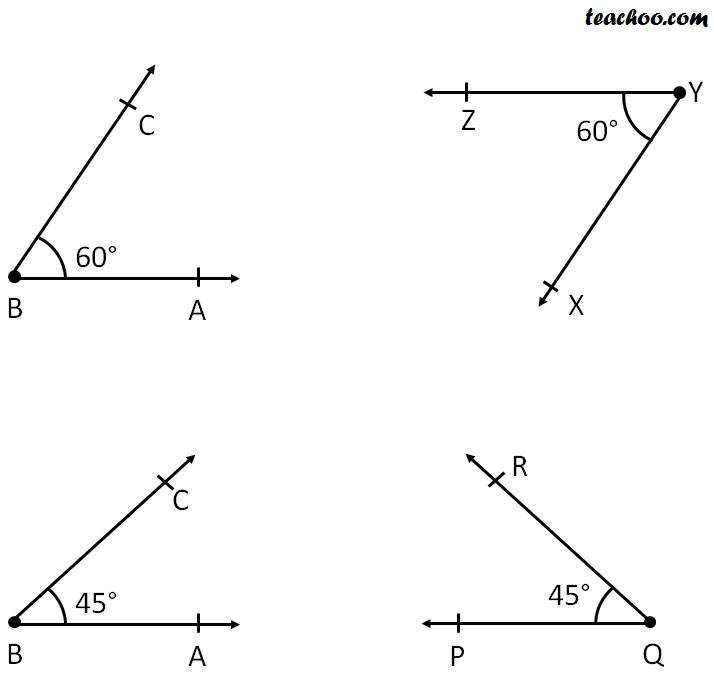Congruency

Chapter 7 Class 7 Congruence of Triangles
Concept wise

Suppose we have two angles

∠ ABC and ∠ PQRTwo angles are congruent if

• They have same shape
• They have same size

Now,

All angles of same value will have same shape.

For example:

∠ABC and ∠PQR have

• Same shape - both are angles
• Same size - Both are 30°

So, they are congruent

So, Angles with same values are congruent

Therefore,

Two angles are congruent if they have the same value .

Other examples can be:Get live Maths 1-on-1 Classs - Class 6 to 12